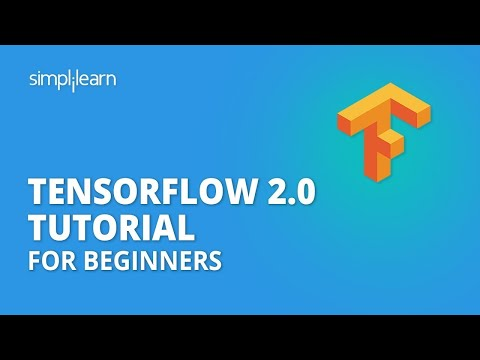Out of the many Deep Learning frameworks available today, TensorFlow is one of the most popular and widely used Deep Learning libraries in the companies these days. It helps you work with complex data and build neural network models to solve business problems. In this article, we’ll learn about what TensorFlow 2.0 really is.

## What Is TensorFlow 2.0?

TensorFlow 2.0 is a library that provides a comprehensive ecosystem of tools for developers, researchers, and organizations who want to build scalable Machine Learning and Deep Learning applications.

TensorFlow is a popular open-source library released in 2015 by the Google Brain team for building machine learning and deep learning models. It is based on Python programming language and performs numerical computations using data flow graphs to build models.

Now that you have understood the ‘what is TensorFlow 2.0’ bit, let’s see the important features of TensorFlow 2.0.

#### Artificial Intelligence Engineer

Your Gateway to Becoming a Successful AI Expert## Features of TensorFlow 2.0

• TensorFlow 2.0 supports easy model building with Keras and eager execution.
• It has robust model deployment in production on any platform.
• You can perform robust experimentation for research.
• TensorFlow 2.0 simplifies the API by cleaning up deprecated APIs and reducing duplication.
• TensorFlow 2.0 works efficiently with multi-dimensional arrays.
• It provides scalability of computation across machines and large data sets.
• TensorFlow 2.0 supports fast debugging and model building.
• It has a large community and provides TensorBoard to visualize the model.

## TensorFlow Applications

Below are a few examples where TensorFlow is being widely used:

• Face detection in electronic devices.
• Machine language translation through apps such as Google Translate.
• Fraud detection in the banking and financial sectors.
• Object detections on videos.

#### FREE Machine Learning Course

Learn In-demand Machine Learning Skills and Tools## What Is a Tensor?

TensorFlow is derived from its core component known as a tensor. A tensor is actually a vector or a matrix of n-dimensions that represents all types of data.In TensorFlow, we define tensors by a unit of dimensionality known as a rank.TensorFlow performs computations with the help of dataflow graphs. It has nodes that represent the operations in your model.

Let’s compute the function depicted below and see how TensorFlow works:

a(b, c, d) = (b + c) * (d - 4)

x = (b + c)

y = (d - 4)

a = x * y

The graph nodes are the inputs and perform mathematical computations, while the connections carry the weights. In this case, it’s the result of an expression.Displayed below is the output:Now, it’s time to learn the major difference between 1.0 and 2.0 versions of TensorFlow in this ‘What is TensorFlow 2.0’ article.

## TensorFlow 1.0 vs 2.0

• TensorFlow 2.0 supports eager execution by default. It allows you to build your models and run them instantly.• Keras is the official high-level API of TensorFlow 2.0. TensorFlow has incorporated Keras as tf.keras. It provides several model building APIs such as Sequential, Functional, and Sub-classing.
• tf.variable_scope is not required in TensorFlow 2.0.• API Cleanup in TensorFlow 2.0• tf.function() and AutoGraph feature## TensorFlow 2.0 Architecture

Over the last few years, the developer community has added many components to TensorFlow. These components will be packaged together into a comprehensive platform that supports machine learning workflows from training through deployment.

Shown below is the new architecture of TensorFlow 2.0:Build deep learning models in TensorFlow and learn the TensorFlow open-source framework with the Deep Learning Course (with Keras &TensorFlow). Enroll now!

## Conclusion

TensorFlow 2.0 is a really powerful framework that helps you create models using vast volumes of data. After reading this article on ‘What is TensorFlow 2.0’, you learned the advancements in TensorFlow 2.0, the various features of TensorFlow 2.0, and the difference between TensorFlow 1.0 vs. 2.0. Finally, you got an idea about TensorFlow 2.0 architecture.Avijeet Biswal